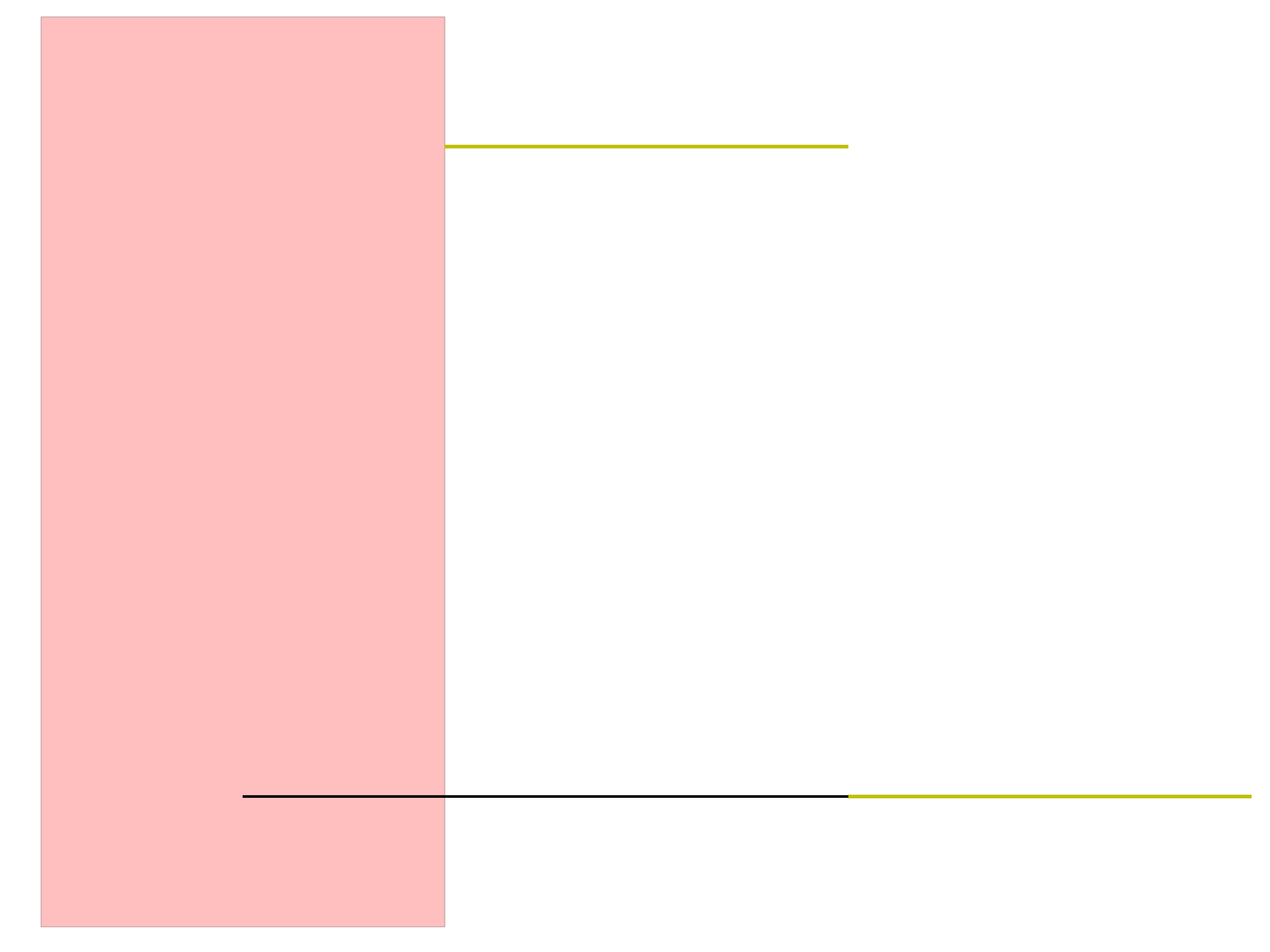LOJ 上有翻译题面

# A. Airport Construction

## 题意

$n\le 200$

## 做法

$k_1\times \overrightarrow{AB}-\overrightarrow{AC}=k_2\times \overrightarrow{CD}$

$k_1=\frac{\overrightarrow{AC}\times \overrightarrow{CD}}{\overrightarrow{AB} \times \overrightarrow{CD}}$

$k_1\times |AB|$$A$ 到交点的有向距离

# B. Get a Clue!

## 题意

$6$ 张写着不同角色的卡， $6$ 张写着不同武器的卡和 $9$ 张写着不同房间的卡

$4$ 个人玩游戏，三种卡各有一张缺失，$1,2$ 号玩家初始得到 $5$ 张卡，$3,4$ 号玩家得到 $4$

$n\le 50$

# C. Mission Improbable

## 题意

$r,c\le 100$

# D. Money for Nothing

## 题意

$n,m\le 5\times 10^5$

# E. Need for Speed

## 题意

$n$ 段行程，现在已知总用时和每段行程的距离 $d_i$ 和速度 $s_i$ 加上常数 $c$ 后的值

# F. Posterize

## 题意

$n$ 个数 $r_1,r_2,\dotsc,r_n$，你需要选出 $k$ 个数 $v_1,v_2,\dotsc,v_k$

$\sum_{i=1}^n \min_{1\le j\le k} (r_i-v_j)^2$

$0\le r_i<256$

dp 即可，两侧的点特判

# G. Replicate Replicate Rfplicbte

## 题意

$w,h\le 300$

# H. Scenery

Tarjan 有论文 = =

## 题意

$n$ 个区间，问是否能选出每个区间的一个长度为 $t$ 的子区间，并且这 $n$ 个子区间不相交（不含端点）

$n\le 10000$

## 做法H-Sample-3

1. 如果 $C<s$，那么不存在方案
2. 如果 $C<s+t$，那么把 $(C-t, s)$ 这个区间标记为 forbidden，即不能被选择，否则 $[s,e]$ 包含的所有区间必然无法同时被满足
3. 如果 $C\ge s+t$，那么不会对 $s$ 及更小的位置造成影响

• 若不存在一个更早选择的子区间，对应的原区间的右端点更大，那么 $[-\infty,e]$ 的区间会在上述的过程中判为不合法
• 若存在，找出一个最靠后的区间，设是第 $j$ 个，那么找出第 $j$ 个到第 $i-1$ 个子区间对应的原区间的左端点的最小值 $s$$[s,e]$ 会在上述过程中被判为不合法

forbidden 区间的右端点都是一个原区间的左端点，根据这个可以简单地 $\mathcal O(n^2)$ 实现

# I. Secret Chamber at Mount Rushmore

## 题意

$m$ 种小写字母间的转化规则，$n$ 次询问两个单词是否可以转化，长度不超过 $50$

$m\le 500,n\le 50$

# J. Son of Pipe Stream

## 题意

$3$ 号点 Flubber 和水的总流量为 $F$$W$，最大化 $F^a W^{1-a}$，其中 $a$ 是一个给定的实数，要求输出每条边的方向及 Flubber 和水的流量

$n\le 200, c_i\le 10, 1\le v\le 10,0.01\le a\le 0.99$

## 做法

$1$$3$ 的最大流为 $F_{\max}$$2$$3$ 的最大流为 $W_{\max}$

（题解说） 事实上这里必然能取到这两个值，考虑构造一组解

$1$ 开始限制最大流量为 $F^*$ 在新图上跑最大流，可以得到 Flubber 每条边的流量，剩余部分即为水的流量

# K. Tarot Sham Boast

## 题意

$s$ 个长度相同的字符集大小为 $3$ 的字符串，要求按照其在长度为 $n$ 的每个位置等概率随机的串中出现概率排序，概率相同按照输入顺序输出

$n\le 10^6, s\le 10$

## 做法

$\sum_{I\subseteq [n-l+1],I\ne \varnothing} (-1)^{|I|+1} P(\text{X 在 I 中的每个位置都出现了})$

• $|I|=1$ 时，概率为 $\frac{n-l+1}{3^l}$，与 $X$ 无关
• $|I|=2$$I$ 中的两个位置相距不小于 $l$，也与 $X$ 无关
• $|I|=2$ 且两个串有交，概率为若干形如 $-3^k$ 的数的和，且 $k$ 两两不同
• $|I|>2$根据题解，可以不考虑 证明在 这里

# L. Visual Python++

## 题意

$n\le 10^5$

0%